## How to convert between date and Unix timestamp in Excel?

Unix timestamp is also called Epoch time or POSIX time which is wildly used in many operating systems or file formats. This tutorial is talking about the conversion between date and Unix timestamp in Excel.

Convert date to timestamp

Convert date and time to timestamp

Convert timestamp to date

####Convert date to timestamp

To convert date to timestamp, a formula can work it out.

Select a blank cell, suppose Cell C2, and type this formula =(C2-DATE(1970,1,1))*86400 into it and press Enter key, if you need, you can apply a range with this formula by dragging the autofill handle. Now a range of date cells have been converted to Unix timestamps.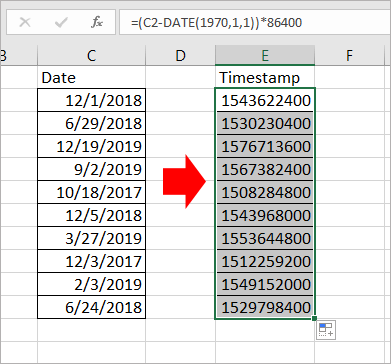### 2 Clicks to Convert Time to Decimal Hours, Minutes or Seconds

The Convert Time feature of Kutools for Excel can help you quickly convert times to decimal hours, minues, or seconds and place the result in the original destination or another one.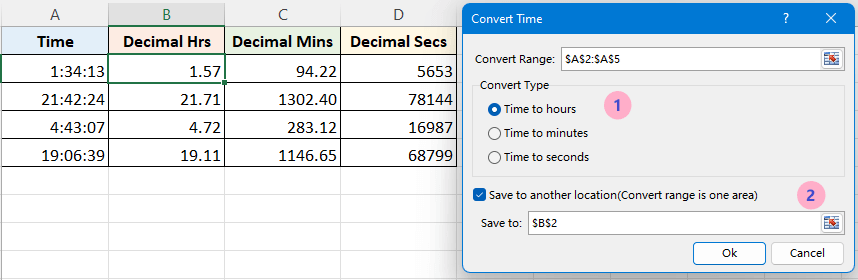####Convert date and time to timestamp

There is a formula that can help you convert date and time to Unix timestamp.

1. Firstly, you need to type the Coordinated Universal Time into a cell, 1/1/1970. See screenshot: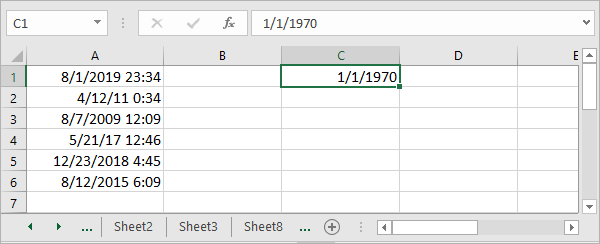2. Then type this formula =(A1-\$C\$1)*86400 into a cell, press Enter key, then if you need, drag the autofill handle to a range with this formula. See screenshot: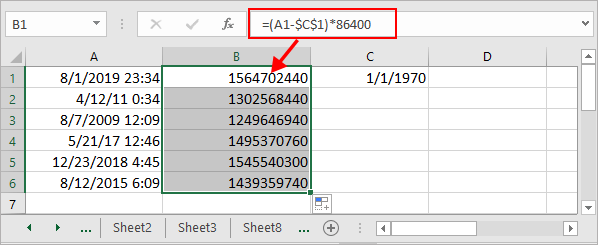Tips: In the formula, A1 is the date and time cell, C1 is the coordinate universal time you typed.

####Convert timestamp to date

If you have a list of timestamp needed to convert to date, you can do as below steps:

1. In a blank cell next to your timestamp list and type this formula =(((A1/60)/60)/24)+DATE(1970,1,1), press Enter key, then drag the auto fill handle to a range you need.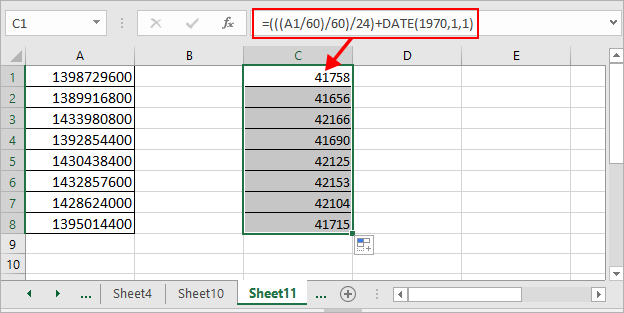2. Then right click the cells used the formula, and select Format Cells from the context menu, then in the popping Format Cells dialog, under Number tab, click Date in the Category list, then select the date type in the right section.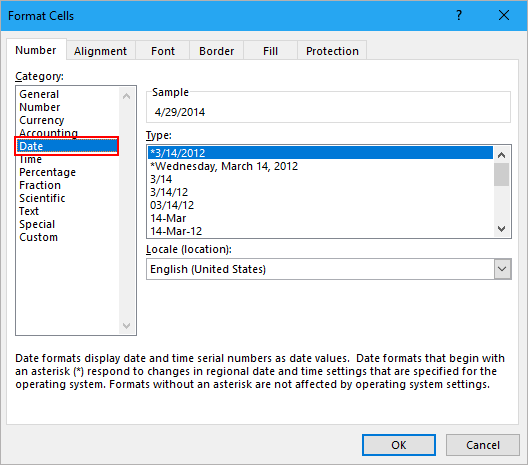3. Click OK, now you can see the Unix timestamps have been converted to dates.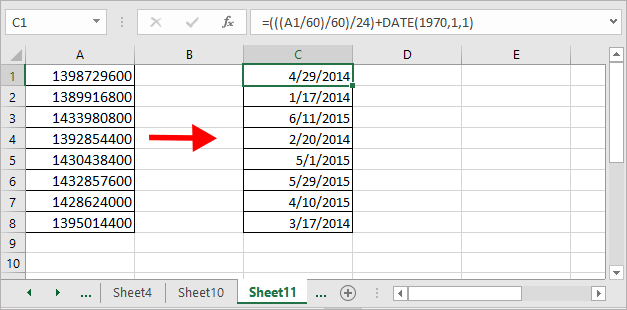Notes:

1. A1 indicates the timestamp cell you need.

2. This formula also can use to convert timestamp series to date and time, just format the result to the date and time format.

3. The above formula converts 10-digits number to a standard datetime, if you want to convert 11-digits number, or 13-digits number, or 16-digits number to a standard datetime in Excel, please use formula as below:

Convert 11-digits number to date: =A1/864000+DATE(1970,1,1)

Convert 13-digits number to date: =A1/86400000+DATE(1970,1,1)

Convert 16-digits number to date: =A1/86400000000+DATE(1970,1,1)

For different lengths of number which needed to be converted to datetime, just change the number of zeros of the divisor in the formula to correctly get the result.

#### Relative Articles:

• How to remove time from date in Excel?
If there has a column of date with time stamp, such as 2/17/2012 12:23, and you don’t want to retain the time stamp and want to remove the time 12:23 from the date and only leave the date 2/17/2012. How could you quickly remove time from date in mulitple cells in Excel?

• How to combine date and time into one cell in Excel?
There are two columns in a worksheet, one is the date, the other is time. Is there any way to quickly combine these two columns into one, and keep the time format? Now, This article introduces two ways in Excel to combine date column and time column into one and keep the time format.

### Best Office Productivity Tools

 Popular Features: Find, Highlight or Identify Duplicates   |  Delete Blank Rows   |  Combine Columns or Cells without Losing Data   |   Round without Formula ... Super Lookup: Multiple Criteria VLookup  |   Multiple Value VLookup  |   VLookup Across Multiple Sheets   |   Fuzzy Lookup .... Advanced Drop-down List: Quickly Create Drop Down List   |  Dependent Drop Down List   |  Multi-select Drop Down List .... Column Manager: Add a Specific Number of Columns  |  Move Columns  |  Toggle Visibility Status of Hidden Columns  |  Compare Ranges & Columns ... Featured Features: Grid Focus   |  Design View   |   Big Formula Bar   |  Workbook & Sheet Manager   |  Resource Library (Auto Text)   |  Date Picker   |  Combine Worksheets   |  Encrypt/Decrypt Cells   |  Send Emails by List   |  Super Filter   |   Special Filter (filter bold/italic/strikethrough...) ... Top 15 Toolsets:  12 Text Tools (Add Text, Remove Characters, ...)   |   50+ Chart Types (Gantt Chart, ...)   |   40+ Practical Formulas (Calculate age based on birthday, ...)   |   19 Insertion Tools (Insert QR Code, Insert Picture from Path, ...)   |   12 Conversion Tools (Numbers to Words, Currency Conversion, ...)   |   7 Merge & Split Tools (Advanced Combine Rows, Split Cells, ...)   |   ... and more

Supercharge Your Excel Skills with Kutools for Excel, and Experience Efficiency Like Never Before. Kutools for Excel Offers Over 300 Advanced Features to Boost Productivity and Save Time.  Click Here to Get The Feature You Need The Most...#### Office Tab Brings Tabbed interface to Office, and Make Your Work Much Easier

• Enable tabbed editing and reading in Word, Excel, PowerPoint, Publisher, Access, Visio and Project.
• Open and create multiple documents in new tabs of the same window, rather than in new windows.
• Increases your productivity by 50%, and reduces hundreds of mouse clicks for you every day!No ratings yet. Be the first to rate!
This comment was minimized by the moderator on the site
How Define a Custom Date Format: yyyy-mm-dd hh:mm:ss

yyyy: Year with four digits.
mm: Month with two digits (01-12).
dd: Day of the month with two digits (01-31).
hh: Hour with two digits (00-23).
mm: Minute with two digits (00-59).
ss: Second with two digits (00-59).
This comment was minimized by the moderator on the site
Hi, Monero Jeanniton, to format a cell with custom date format, just right-click on the cell, select Format Cells, then type the format into Type of Custom section. See screenshot: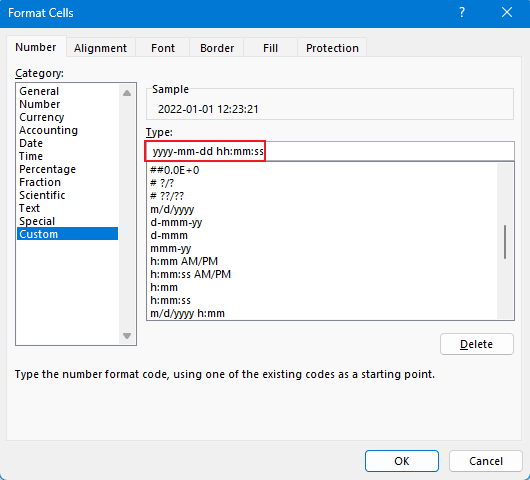This comment was minimized by the moderator on the site
Converted 123000 Lines of Data Stamp 13 Digits like a PRO Thanks alot for the tips
This comment was minimized by the moderator on the site
I have a numeric value of 3721719999 which is suppose to display as 00:06:12 (hh:mm:ss). This time represents the time duration of a video file. The date stamp on the file name is 1/18/2014 4:04 pm, if that offers any further information. I extracted the number using Filelist.exe and this was the result. Thanks!
This comment was minimized by the moderator on the site
How to convert 13 digits timestamp to human readable date ?
This comment was minimized by the moderator on the site
Hi, Roobanraj, to convert 13 digits timestamp to a standard datetime, you can use the formula as this:=A1/86400000+DATE(1970,1,1), A1 is the cell that contains the 13-digits you want to convert to.
This comment was minimized by the moderator on the site
How to convert 11 digits timestamp to human readable date ?
This comment was minimized by the moderator on the site
For converting 11 digits timestamp to a standard datetime, use the formula as this:=A1/864000+DATE(1970,1,1), A1 is the cell that contains the 11-digits you want to convert to.
This comment was minimized by the moderator on the site
how to convert event time stamp to hour
This comment was minimized by the moderator on the site
To convert date for MOODLE the only formula that worked was:

I tried 20/october/1980 and Moodle read 19/october/1980.

I used
=(C2-DATE(1970,1,0))*86400

I tried 20/october/1980 and Moodle read 20/october/1980.
This comment was minimized by the moderator on the site
How to convert 21 digits timestamp to human readable date ?
E.g., 202002191100506253230
This comment was minimized by the moderator on the site
IF you have an LDAP 18-Digit timestamp such as 132079258679926000 the below formulas will not work. You first have to convert them as they are based in nano seconds from 1/1/1601. The following formula in Excel converts the LDAP to EPOCH to human dates =(((((A1/10000000)-11644473900)/60)/60)/24)+DATE(1970,1,1) where A1 refers to your timestamp location
This comment was minimized by the moderator on the site
This comment was minimized by the moderator on the site
One thing to note is that the above is for epoch time in seconds. If you need milliseconds, you need to add a further multiplication / division by 1000. For example, converting from epoch time (milliseconds) to a date would be "=((((A1/1000)/60)/60)/24)+DATE(1970,1,1)".
This comment was minimized by the moderator on the site
Muchas gracias, para mi caso estaba adivinando porque no conocía que tipo de dato venia pero este fue la solución, el dato que me pasaron era con esta forma 1641014648299 y la formula fue esta =((((C2/1000)/60)/60)/24 )+FECHA(1970;1;1) por lo cual si alguien esta en un caso similar al mío puede optar por esta formula.

Esta formula también me sirvió pero solo trae fecha =A1/86400000+FECHA(1970;1;1)
This comment was minimized by the moderator on the site
As a side note, the best way to tell if it's seconds or milliseconds is looking at the length of the field; if it's 10 digits, it's seconds, and if it's 13 digits, it's milliseconds. "=LEN(A1)" will tell you how long the value is.
This comment was minimized by the moderator on the site
If that's too much manual work for you (or you have a list that's mixed between seconds and milliseconds), then you can use the following formula to automatically switch between seconds and milliseconds based on the length of the cell: "=((A1/86400)/IF(LEN(A1)=13,1000,1))+DATE(1970,1,1)".
There are no comments posted here yet